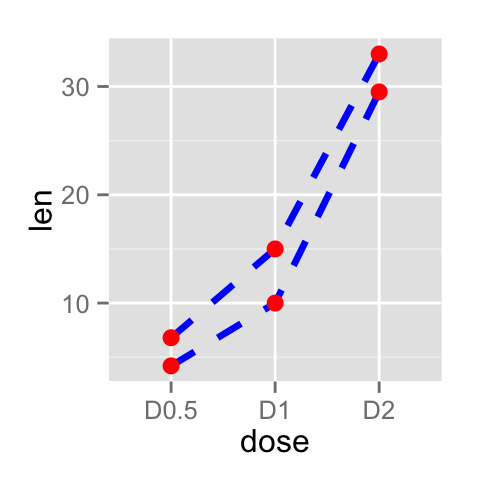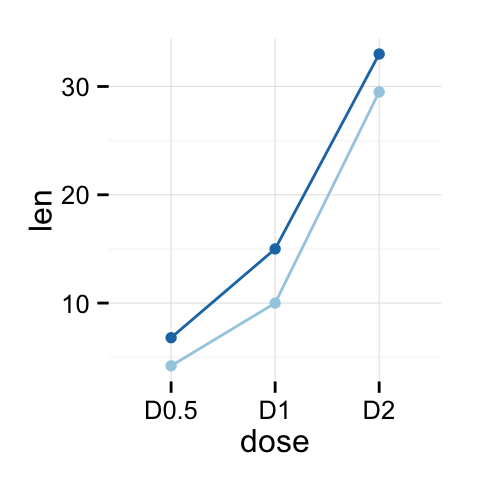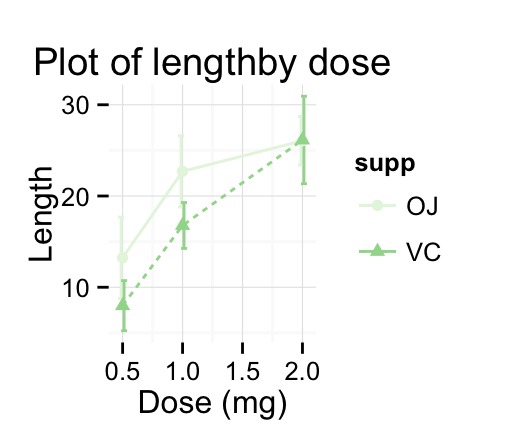This R tutorial describes how to create line plots using R software and ggplot2 package.

In a line graph, observations are ordered by x value and connected.

The functions geom_line(), geom_step(), or geom_path() can be used.

x value (for x axis) can be :

• date : for a time series data
• texts
• discrete numeric values
• continuous numeric values

•# Basic line plots

## Data

Data derived from ToothGrowth data sets are used. ToothGrowth describes the effect of Vitamin C on tooth growth in Guinea pigs.

• len : Tooth length
• dose : Dose in milligrams (0.5, 1, 2)

• ## Create line plots with pointsRead more on line types : ggplot2 line types

You can add an arrow to the line using the grid package :Observations can be also connected using the functions geom_step() or geom_path() :• geom_line : Connecting observations, ordered by x value
• geom_path() : Observations are connected in original order
• geom_step : Connecting observations by stairs

• # Line plot with multiple groups

## Data

Data derived from ToothGrowth data sets are used. ToothGrowth describes the effect of Vitamin C on tooth growth in Guinea pigs. Three dose levels of Vitamin C (0.5, 1, and 2 mg) with each of two delivery methods [orange juice (OJ) or ascorbic acid (VC)] are used :

• len : Tooth length
• dose : Dose in milligrams (0.5, 1, 2)
• supp : Supplement type (VC or OJ)

• ## Create line plots

In the graphs below, line types, colors and sizes are the same for the two groups :## Change line types by groups

In the graphs below, line types and point shapes are controlled automatically by the levels of the variable supp :It is also possible to change manually the line types using the function scale_linetype_manual().You can read more on line types here : ggplot2 line types

## Change line colors by groups

Line colors are controlled automatically by the levels of the variable supp :It is also possible to change manually line colors using the functions :

• scale_color_manual() : to use custom colors
• scale_color_brewer() : to use color palettes from RColorBrewer package
• scale_color_grey() : to use grey color palettes

•Read more on ggplot2 colors here : ggplot2 colors

# Change the legend positionThe allowed values for the arguments legend.position are : “left”,”top”, “right”, “bottom”.

Read more on ggplot legend : ggplot2 legend

# Line plot with a numeric x-axis

If the variable on x-axis is numeric, it can be useful to treat it as a continuous or a factor variable depending on what you want to do :# Line plot with dates on x-axis

economics time series data sets are used :

Plots :Change line size :# Line graph with error bars

The function below will be used to calculate the mean and the standard deviation, for the variable of interest, in each group :

Summarize the data :

The function geom_errorbar() can be used to produce a line graph with error bars :# Customized line graphsChange colors manually :# Infos

This analysis has been performed using R software (ver. 3.1.2) and ggplot2 (ver. 1.0.0)

Contribution from ：http://www.sthda.com/english/wiki/ggplot2-line-plot-quick-start-guide-r-software-and-data-visualization欢迎关注
0%